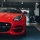Home
IT Knowledge
Inspiration
Languages
EN

# PostgreSQL - ROUND() function example

0 points
Created by:Explosssive
309

In this article, we would like to show you how to use `ROUND()` function in PostgreSQL.

Quick solution:

``SELECT ROUND(number, decimals);``

## Practical example

### Simple example

In this example, we will show you how to simply round a number to four decimal places using `ROUND()` function.

Query:

``SELECT ROUND(100.12345, 4);``

Output:

``100.1235``

To show more complex example of how the `ROUND()` function works, we will use the following table:

Note:

At the end of this article you can find database preparation SQL queries.

### Example 1

In this example, we will round the salary column to 0 decimal places.

Query:

``````SELECT ROUND("salary", 0)
FROM "users";``````

Output:

### Example 2

In this example, we will display the average salary rounded to 2 decimal places.

Query:

``````SELECT ROUND(AVG("salary"), 2)
FROM "users";``````

Output:

## Database preparation

`create_tables.sql` file:

``````CREATE TABLE "users" (
"id" SERIAL,
"name" VARCHAR(50) NOT NULL,
"surname" VARCHAR(50) NOT NULL,
"department_id" INTEGER,
"salary" DECIMAL(15,2) NOT NULL,
PRIMARY KEY ("id")
);``````

`insert_data.sql` file:

``````INSERT INTO "users"
( "name", "surname", "department_id", "salary")
VALUES
('John', 'Stewart', 1, '3512.00'),
('Chris', 'Brown', 2, '1344.00'),
('Kate', 'Lewis', 3, '6574.00'),
('Ailisa', 'Gomez', NULL, '6500.00'),
('Gwendolyn', 'James', 2, '4200.00'),
('Simon', 'Collins', 4, '3320.00'),
('Taylor', 'Martin', 2, '1500.00'),
('Andrew', 'Thompson', NULL, '2100.00');``````